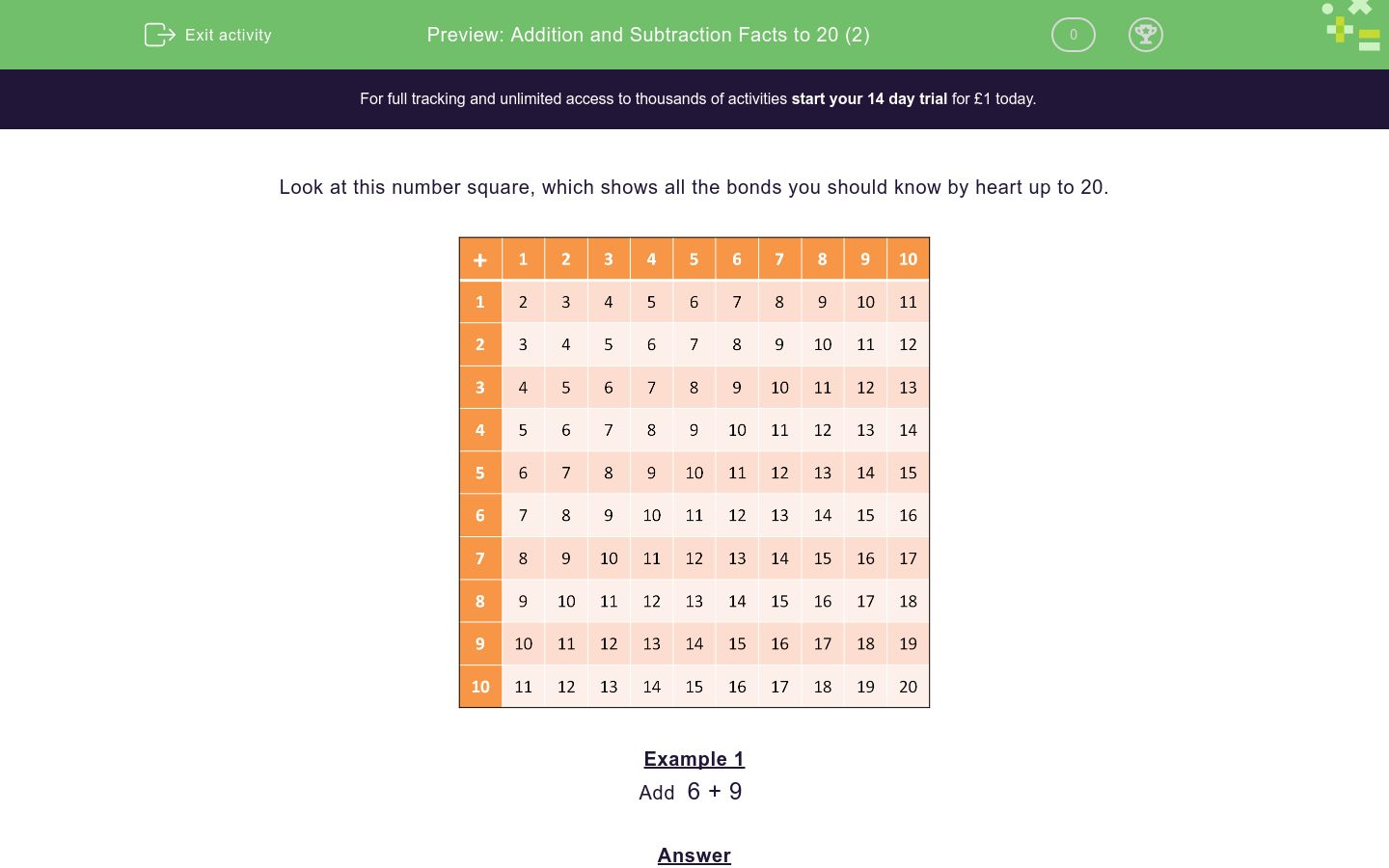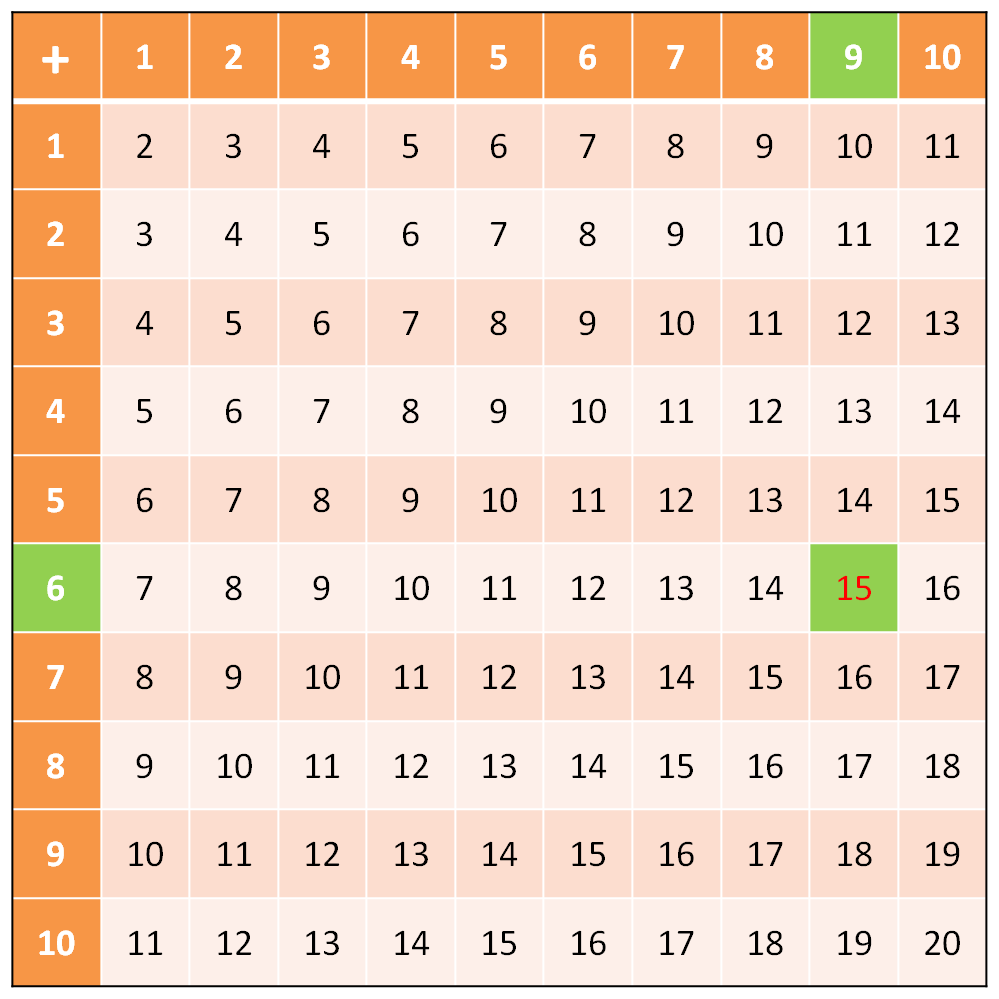# Addition and Subtraction Facts to 20 (2)

In this worksheet, students must know by heart their addition and subtraction number bonds to 20. No explanations are given.Key stage:  KS 1

Curriculum topic:   Number: Addition and Subtraction

Curriculum subtopic:   Use Addition/Subtraction Facts to 100

Difficulty level:#### Worksheet Overview

Look at this number square, which shows all the bonds you should know by heart up to 20.Example 1

You should know this by heart, but the number square shows that it is 15.Example 2

Subtract  15 - 9

You should know this by heart, but the number square shows that it is 6.

When you subtract, the starting number is inside the square.Example 3

You should know that   2 + 5 = 7,

so 12 + 5 = 17

Example 4

Subtract 16 - 12